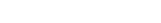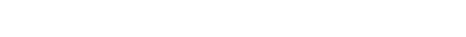## Chemistry Paper 1 Questions - Maseno Mock Examinations 2022

QUESTIONS

1.
1. A student assigns the name butan-3-ol to
CH3CH2-CHCH3
I
OH
Why is this incorrect?(1mk)
2. The organic compound in 1 (a) above is heated with Aluminium oxide at 300ᵒC. Draw the structural formulae of the two organic compounds that would be produced. (1mk)
2. Study the scheme below and answer the questions that follow.1. Carbon (II) Oxide is main reducing agents. Show how it is obtained in this process.(1mk)
2. Usually zinc is found mixed with lead ore. What property of zinc makes it possible to separate it from its mixture? (1mk)
3. Zinc is used to make brass. Give any one use of brass. (1mk)
3. The following data was obtained in an experiment to determine the enthalpy of combustion of propanol via heats of formation.
Equation                                              Enthalpy of formation
H2(g) + ½O2(g) → H2O(g)                       ΔH1 = -286kJMol
C(S)+ O2(g) → CO2(g)                           ΔH2 = -393kJMol
3C(S) + H2(g) + ½O2(g) → C3H7OH      ΔH3 = -307kJmol
1. State Hess’ law of constant heat summation. (1mk)
2. Calculate the molar enthalpy of combustion of propanol. (2mks)
4. The graph bellow shows how the pressure of fixed mass of a gas varies with its absolute temperature.1. Explain the variation of pressure with absolute temperature for a fixed mass of a gas. (1mk)
2. If the volume of a sample of chlorine gas is 8.2L at 1.2atm and 33°C, what volume will the gas occupy at S.T.P (temperature = 0°C, pressure =1atm) (2mks)
5.
1. Equal volumes of all gases under the same conditions of temperature and pressure contain the same number of molecules. Which law is this? (1mk)
2. Calculate the number of ammonium ions in 250cm³ of 0.5M ammonium phosphate solution. (1 mole=6.022×10²³ ions) (2mks)
6. Two cleansing agents are represented by the formula
X - RCOO-Na+1. Name the class of cleansing agent to which RCOO-Na+belongs. (1mk)
2. Which cleansing agents would be suitable for use in water containing dissolved calcium chlorate (I)? Explain. (1mk)
7. The diagram below shows the apparatus used to prepare dry gas XThe gas X produced in the flask turned filter paper soaked in lead (II) ethanoate solution black. The solutions obtained in the reaction formed a white precipitate soluble in excess alkali of ammonia solution.
1. Complete the diagram to show how the dry gas would be collected. (1mk)
2. Write an equation for the formation of the black substance. (1mk)
3. Name the cation formed when the white precipitate dissolved in excess aqueous ammonia. (1mk)
8. In an experiment dry hydrogen gas was passed over heated metal oxide as shown in the diagram below.1. Explain the necessary precaution at the end of this experiment. (1mk)
2. State the observation made at the point where excess hydrogen burns. (1mk)
3. Name a suitable chemical substance that can be used in the chemical test for the product formed when hydrogen burns. (1mk)
9. State and explain the change in mass that occurs when potassium manganate (VII) is heated strongly in an open crucible. (2mks)
10. Complete the table below for the sub-atomic particles. (2mks)
 Particle Proton Neutron Electrons 20782Pb4+ 82 3115P2-
11. A current of 2.0 Ampere was passed through dilute silver nitrate solution for 20 minutes. Determine the volume of the gas liberated at the anode. (Molar gas volume is 24000cm³ Ag=108, 1Faraday= 96500coloumbs) (2mks)
12.
1. What is meant by a reversible reaction. (1mk)
2. In the haber process nitrogen and hydrogen react according to the equation
3H2(g) + N2(g) → 2NH3(g) ΔH= -ve.
State and explain the effect of adding hydrogen chloride gas on the position of equilibrium. (2mks)
13.
1. During preparation of ammonia gas using Ca(OH)2, why should the apparatus be slanted? (1mk)
2. Under what conditions does ammonia gas burn in air? (2mks)
14. The set up below shows the apparatus used to determine the percentage of oxygen in the Air.The air was passed through the heated copper turning slowly and repeatedly until constant volume of air was obtained.
1. What is the role of the glass wool. (1mk)
2. State the observation made at the end of the experiment if copper was replaced with lead. (1mk)
3. State one possible source of error in this experiment. (1mk)
15. The following data gives pH values of solutions P, Q, R, S, and T
 Solution P Q R S T pH value 8.5 5.5 7.0 1.5 13.6
1. Which solution would be that of milk of magnesia? (1mk)
2. What would be the colour of solution R after adding a few drops of screened methyl orange indicator. (2mks)
3. In which solutions would Aluminium hydroxide dissolve? Give a reason. (1mk)
16. Write equation to show the effects of heat on;
1. Silver carbonate. (1mk)
2. Aluminium nitrate. (1mk)
3. Anhydrous iron (II) sulphate. (1mk)
17.
1. Chlorine gas is bubbled through an aqueous solution of potassium iodide in a test tube. Give the formula of the ion expected. (1mk)
2. What is observed when aqueous silver nitrate solution is added to an aqueous solution of potassium iodide? (1mk)
3. Write an ionic equation for the reaction in (b) above. (1mk)
18. The table below gives reduction potentials obtained when half-cells are connected to a standard hydrogen electrodes
Electrode reactions        E°volt
Mg²+(aq) +2e  Mg(s)   -2.37V
Cd²+(aq) + 2e ⇌ Cd(s)   -0.42V
Zn²+(aq) +2e ⇌ Zn(s)     -0.76V
Ag+(aq) + e ⇌ Ag(s)      +0.80V
Cu²+(aq) + 2e ⇌ Cu(s)   +0.34V
1. Choose the half cells giving the least electrode potential when combined. (1mk)
2. Can the reaction 2Ag(s) + Cu²+(aq) 2Ag+(aq) + Cu(s) take place? Show your working. (2mks)
19. Below is part of a radioactive decay series.1. What is meant by radioactive decay series. (1mk)
2. Write down the nuclear equation for the reaction that converts 22888Ra to 22086Rn. (1mk)
3. State any one use of radio-isotopes in agriculture. (1mk)
20. Using dots (.) and crosses(x) to represent the electrons show bonding in a compound formed between.
1.
1. Magnesium and flourine (Mg = 12.0 F= 9.0) (1mk)
2. Carbon and flourine (C= 6.0, F=9.0) (1mk)
2. In terms of structure and bonding. Explain why Dimethyl ether (CH3-O-CH3) boils at -24°C while ethanol (CH3CH2OH) boils at 78°C even though the two compounds have the same molar mass. (1mk)
21. Describe how you would proceed to separate a mixture of Aluminium chloride, silver chloride and Magnesium chloride. (3mks)
22. Two organic compounds are represented by thecondensed structural formulae as shown bellow.
1. CH3CH2C(CH3)2CHCH2
2. CH3CH2CH2CH2OH
1. The organic compound represented as I above is shaken with bromine water identify the products formed. (1mk)
2. Write a balanced equation for the complete combustion of the organic compound represented in II above. (1mk)
3. Give any one use of CH3CH2CH2CH2OH. (1mk)
23. The table below shows some properties of some elements in period 3 of the periodic table.
 Element Sodium Magnesium Aluminium Melting point 97.8 650 660 Atomic radii (nm) 0.152 0.136 0.125
1. Explain the trend of the melting points of the elements. (2mks)
2. Why is there a decrease in size of atoms from sodium to aluminium? (1mk)
24. The graph below shows a curve obtained when water at 20°C was heated for 20 minutes.1. What happens to water molecules between point W and X. (1mk)
2. In which part of the curve does the change of state occur. (1mk)
3. Explain why the temperature does not rise between points X and Y. (1mk)
25. Starting with 120cm³ of 3M phosphoric (V) acid, describe how you would proceed to prepare pure crystals of potassium phosphate. (3mks)
26. 0.24g of divalent metal X was dissolved in 50cm³ of 0.25M sulphuric (VI) acid. The resulting solution required 5cm³ of 1M sodium hydroxide solution to neutralize the excess acid. What is the Relative atomic Mass of X. (3mks)
27. Diamond and graphite are both allotropes of carbon.
1. Define the term allotropes. (1mk)
2. Explain in terms of structure and bonding why diamond is used as an abrasive. (1mk)
3. Describe how carbon (IV) oxide can be distinguished from carbon (II) oxide using lime water. (1mk)
28. A section of polymer is given below. Study it and answer the questions that follow.1. Give the systematic name of the polymer. (1mk)
2. Draw the structure of the repeat unit of the above polymer. (1mk)
3. Give one use of the polymer. (1mk)
29.
1. What is meant by the term electrode? (1mk)
2. A substance Q in molten state conducts electric current and a brown gas is produced at the anode. Name the type of structure present in substance Q. (1mk)

• ✔ To read offline at any time.
• ✔ To Print at your convenience
• ✔ Share Easily with Friends / Students